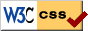# Basic Analysis II: Introduction to Real Analysis, Volume II: Changes

I preserve numbering as much as possible. So for example exercises are only added with new numbers, so that old exercises are not renumbered, etc. I try to preserve pagination as well though adding a page in the middle is sometimes unavoidable.

June 10th 2020 edition, Version 2.3 (edition 2, 3rd update):

1. Improve the introduction.
2. In the statement of inverse function theorem, remove the definition of $q$, it is not used.
3. In proof of second part of 10.4.3 (Riemann-Lebesgue) use $S_{1/k}$ rather than $S_k$ for consistency with the first part of the proof.
4. In the path integral chapter, consistently use the words "path integral" rather than sometimes "path integral" sometimes "line integral".
5. Mention that the nowhere differentiable function construction that we gave is due to Takagi (although it is the one given in Rudin, not the form of it usually attributed to Takagi).
6. Greatly simplify the function in Example 11.6.4.
7. In Exercise 11.6.1, the question should ask about there being no subsequence that converges uniformly just like 11.6.2. The question is about why Arzelà-Ascoli fails.
8. Many minor language and style improvements as well as some minor clarifications.
9. Fix the errata from the last revision.

May 15th 2019 edition, Version 2.2 (edition 2, 2nd update):

1. Several minor grammar and style fixes.
2. Fix the errata from the last revision.

October 11th 2018 edition, Version 2.1 (edition 2, 1st update):

2. Fix errata.

May 7th 2018 edition, Version 2.0 (edition 2, 0th update):

Numbering of definitions, examples, propositions changed in 8.1, 8.3, 10.1. Numbering of exercises is unchanged, except for 9.1.7 which was replaced due to erratum.

1. New section 10.7 on change of variables.
2. New chapter 11 on Arzela-Ascoli, Stone-Weierstrass, power series, and Fourier series.
3. A List of Notations is added at the back as in volume I.
4. In the PDF the pages have been made slightly longer so that we can lower the page count to save some paper.
6. Add a paragraph about simple algebraic facts such that $0v=v$.
8. Add example that span of $t^n$ is ${\mathbb{R}}[t]$.
10. We also use the words "linear operator" for $L(X,Y)$, and it is for $L(X)$ that we say "linear operator on $X$", so update the definition appropriately.
11. Add convexity of $B(x,r)$ as a proposition since we use it so often.
13. Proposition 8.2.4 doesn't need $Y$ to be finite dimensional, same in the exercise 8.2.12, so no need to assume it.
14. In Proposition 8.2.5, emphasize where the finiteness of dimension is needed.
15. Use $GL(X)$ as notation for invertible linear operators.
16. Give more detail on why mapping between matrices and linear operators is one to one once a basis is fixed.
17. Add a commutative diagram to the independence on basis discussion.
18. Reorder the definition of sign of a permutation to be more logical.
19. Add short example of permutation as transpositions.
20. Add exercises 8.2.14, 8.2.15, 8.2.16, 8.2.17, 8.2.18, 8.2.19
21. Add figure to definitions 8.3.1 and 8.3.8.
22. Add Proposition 8.3.6, which was conspicuously missing.
23. Add figure for differentiable curve and its derivative.
24. Add figure to exercises 8.3.5 and 8.3.6.
26. Add graph to figure in example 8.4.3 (and adjust the formulas)
27. As application of continuous partials imply $C^1$
28. Add exercises 8.4.7, 8.4.8, 8.4.9, 8.4.10
29. Fix up statement of the inverse function theorem in 8.4.
30. Add a couple of figures to proof of the inverse function theorem.
31. Add a figure to the implicit function theorem.
33. make the remark at the end of 8.5 into an actual "remark"
34. Add observation about solving a bunch of equations not just for $s=0$ for the implicit function theorem.
35. Add exercises 8.5.9, 8.5.10, 8.5.11
37. In 8.6 cleanup the argument in the proposition and use only positive $s$ and $t$ for simplicity.
38. Add exercises 8.6.5, 8.6.6, 8.6.7
39. Refer to the new proposition 7.5.12 about the continuity in 9.1
40. Add figure to example in 9.1
41. Exercise 9.1.7 replaced due to erratum. The replacement shows the same issue that the previous wrong exercise tried to.
43. Reorder the introduction of 9.2 a bit, and fix an erratum in that derivative at the endpoints was not really defined for mappings.
44. Add figure to examples 9.2.2, 9.2.3, 9.2.11, 9.2.13, 9.2.18
45. Add figure for definition of a function against arc-length measure.
46. Add figure to proof of path independence implies antiderivative in 9.3.
47. Add figure to proof that integral over closed paths being zero means that the integral is path independent in 9.3.
48. Add figure to definition 9.3.5
49. Change hint to exercise 9.3.8.
50. Add Example 10.1.16 of compact support with a figure, following examples/propositions in 10.1 are renumbered.
51. Explicitly mention monotonicity of outer measures right after the definition (it is a rather easy exercise), and also allowing finite sequences of rectangles in the definition (a new exercise).
52. Add figure to definition of outer measure.
53. Clean up proof of Proposition 10.3.2.
54. Add exercises 10.3.11, 10.3.12 (and a figure), 10.3.13.
55. Add corollary for the Riemann integrability theorem showing that it is an algebra, that min and max of two functions are Riemann integrable and so is the absolute value.
56. Add exercises 10.4.6, 10.4.7, 10.4.8, 10.4.9, 10.4.10, 10.4.11
57. Add exercises 10.5.5, 10.5.6, 10.5.7
58. In 10.6, add figure for positive orientation and a figure illustrating the three types of domains.
59. Many minor improvements in style and clarity, plus several small new example throughout.
60. Fix errata.

March 21st 2017 edition, Version 1.0 (edition 1, 0th update):

First version.